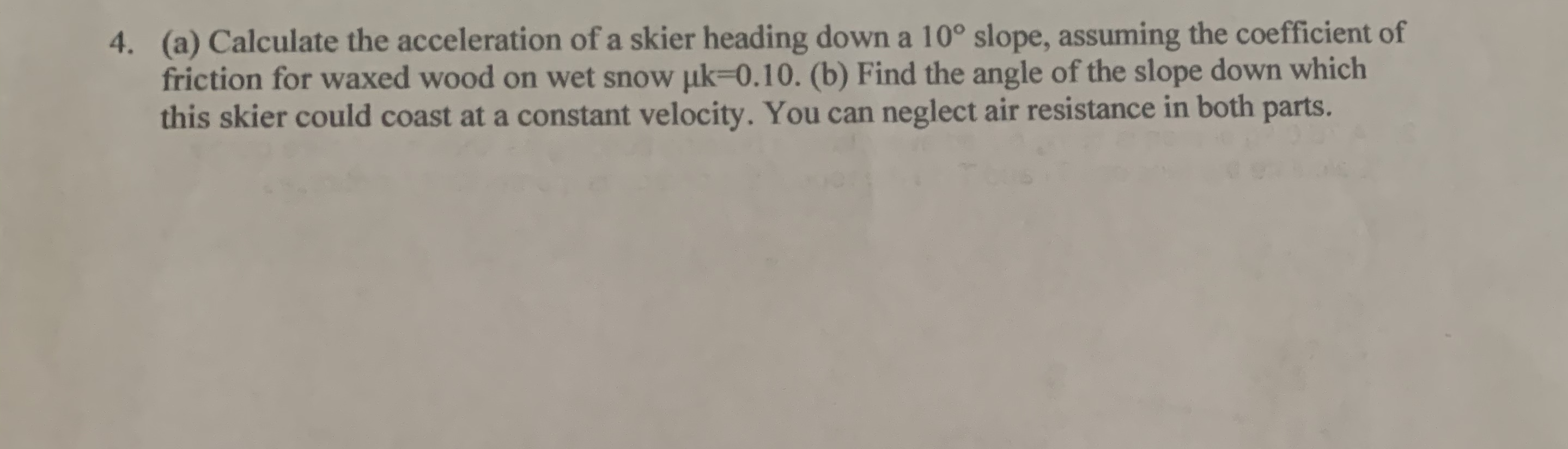# (a) Calculate the acceleration of a skier heading down a 10° slope, assuming the coefficient offriction for waxed wood on wet snow uk-0.10. (b) Find the angle of the slope down whichthis skier could coast at a constant velocity. You can neglect air resistance in both4.parts.

Question
121 viewshelp_outlineImage Transcriptionclose(a) Calculate the acceleration of a skier heading down a 10° slope, assuming the coefficient of friction for waxed wood on wet snow uk-0.10. (b) Find the angle of the slope down which this skier could coast at a constant velocity. You can neglect air resistance in both 4. parts. fullscreen
check_circle

Step 1

(a) Consider the free body diagram below.

Step 2

Using Newton’s law, the acceleration is obtained as,

Step 3

(b) At constant velocity, acceleration is zero. Using the e...

### Want to see the full answer?

See Solution

#### Want to see this answer and more?

Solutions are written by subject experts who are available 24/7. Questions are typically answered within 1 hour.*

See Solution
*Response times may vary by subject and question.
Tagged in

### Kinematics